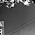# Programming Videos

## Search

### C, C++ Program to Check whether an Input Number is a Perfect Square or not

Write a C, C++ program to check whether an input number is a perfect square or not. In this program, We will not use an in-built function such as sqrt() to solve this question.

C, C++ programming questions for practice

Find cube of a number

Perfect Square

A number is a perfect square if it is the square of an integer.

For example - 25 is a perfect square. You can write it as 5 * 5 which is the square of an integer. But 17 is not a perfect square.

## C Program to Check an Input Number is Perfect Square or not

```#include <stdio.h>

int main(void) {

int num, flag = 0;

printf ("Enter a number \n");
scanf ("%d", &num);

for (int i = 1; i <= num; i++) {

if ( i * i == num) {

flag = 1;
break;
}

if ( i * i > num) {

break;

}
}

if (flag) {

printf (" %d is a perfect square", num);

} else {

printf (" %d is not a perfect square", num);
}

return 0;
}

```

Output :

Enter a number :  4

4 is a perfect square

Enter a number : 7

7 is not a perfect square

## C++ Program to Check wether an Input Number is a Perfect Square or not

```#include <iostream>
using namespace std;

int main() {

int num, flag = 0;

cout << "Enter a number \n";
cin  >> num;

for (int i = 1; i <= num; i++) {

/* If it is equal to an input number
* then it's a perfect square
*/
if ( i * i == num) {

flag = 1;
break;
}

/**
* If it's greater than input number
* so no need to check further
* break the loop
*/
if ( i * i > num) {

break;

}
}

if (flag) {

cout << num << " is a perfect square";

} else {

cout << num << " is not a perfect square";
}

return 0;
}
```

1.2.3.